Latest Teaching jobs   »   Mensuration – 2D : : Download...

# Mensuration – 2D : : Download Mathematics Study Notes Free PDF For REET/UTET Exam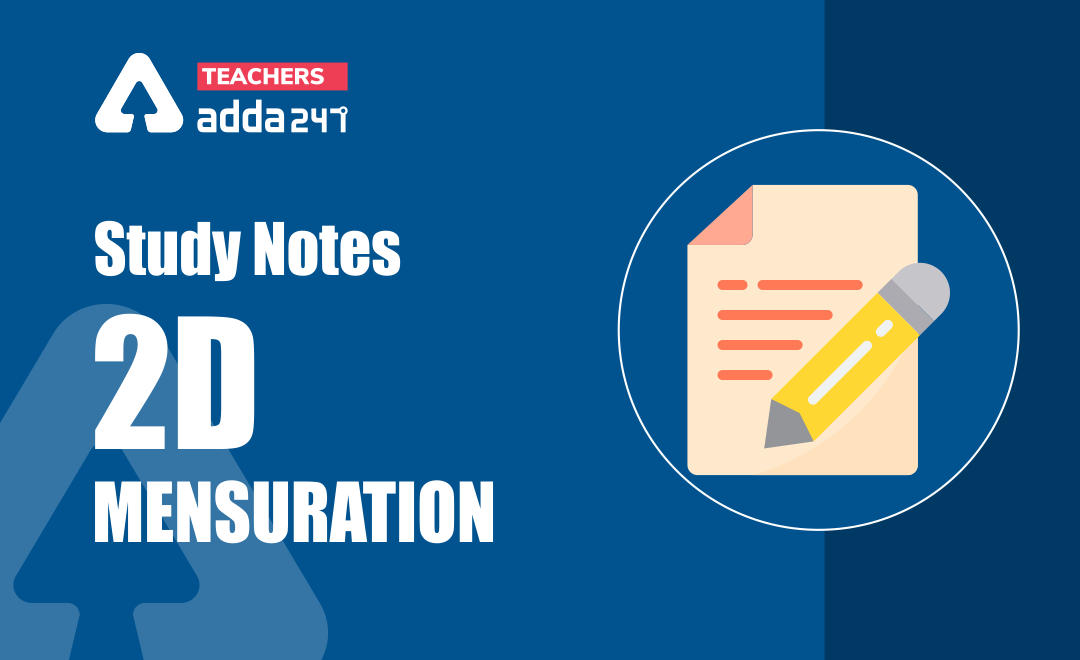## Study Notes on 2D Mensuration

Mathematics is an equally important section for  REETMPTET & DSSSB Exams and has even more abundant importance in some other exams conducted by central or state govt. Generally, there are questions asked related to basic concepts and formulas of the Mensuration.

To let you make the most of Mathematics section, we are providing important facts related to the 2D Mensuration. At least 3-4 questions are asked from mensuration topic in most of the teaching exams. We wish you all the best of luck to come over the fear of the Mathematics section.

How to Overcome Exam Fever, Especially When You Fear Maths

### Rectangle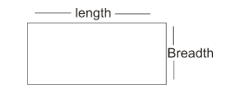(a) Its diagonals are equal and bisect each other

(b) Area = L×B

(c) Diagonal d=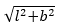(d) (i) Area of a path inside a rectangular field:

Area of Path= 2x(l+b-2x)

(ii) Perimeter (p)= inner p+ outer p

= 2(l+b)+2(l+b-4x)

= 4(l+b-2x)

(e) Room as a Rectangular figure:

Area of 4 walls of a room= perimeter × height

= 2(l+b)×h

(f) Area of roof and 4 walls = 2H (L+B)+LB

Example: A rectangular piece is 40cm long and 30m wide from its four corners, quadrants of radii 3.5m have been cut. The area of remaining part is:

Sol. Area of Rectangle – 4 × area of quarter circle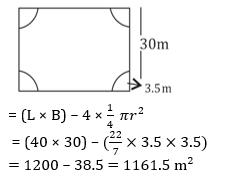### Square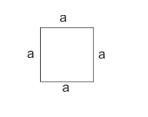(a) Area = a² or ×(diagonal)²

(b) Perimeter = 4a

(c) Diagonal =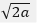(d) Area of path inside square = 4d(x-d)

X= length of square, d= length of path

(e) Area of path outside square = 4d (x+d)

(f) Area of path midway square = d(2x-d)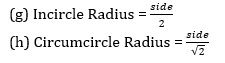Example: If each side of square park is increased by 35%. Find the percentage change in its area?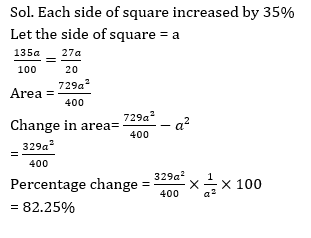Practice REET Previous Year Maths Quiz For REET Exam Here

### Parallelogram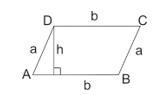(a) Area = base × height

(b) Perimeter= 2(a + b)

(c) d₁²+ d₂²= 2(a²+b²)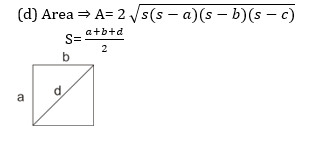Example: A parallelogram PQRS has side PQ = 36cm and PS = 24cm. The distance between the sides PQ and RS is 16cm. Find the distance between the side PS and RQ.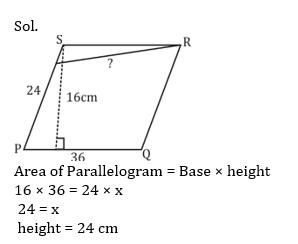Mathematics Study Notes For All Teaching Exams

### Rhombus:

`It is a quadrilateral whose all four sides are equal. Diagonal bisect each other at 90°`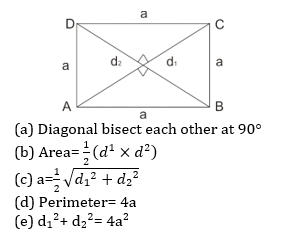Example: The perimeter of Rhombus is 60cm and the measure of an angle is 60° then the area is: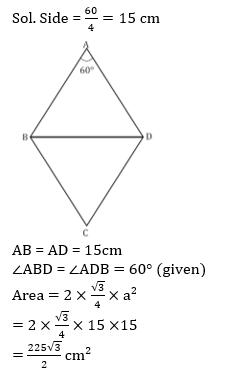### Trapezium

It is a quadrilateral, whose any two opposite sides are parallel.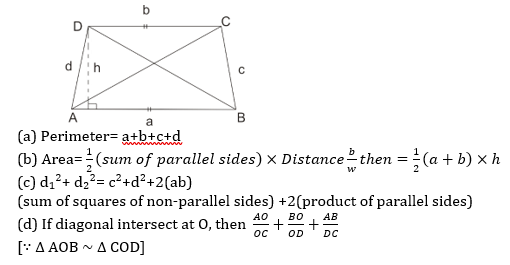Practice Mensuration 2D Quiz Here for CTET Exam

### Triangle

A triangle is a polygon with three edges and three vertices.

Types of triangles:

• Scalene Triangle: A scalene triangle has all its sides of different lengths.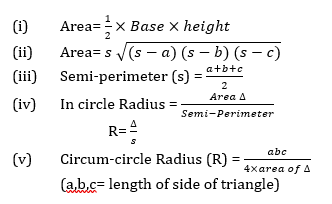Example: The ratio of sides of a triangle is 4:5:6. If perimeter of triangle is 90 cm then the Area of triangle is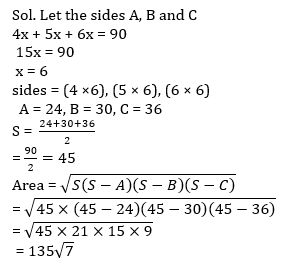• Isosceles Triangle: An Isosceles triangle is a triangle with two equal sides also their opposite angles are equal.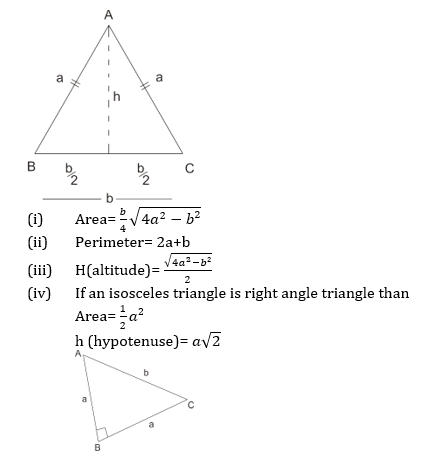• Right angle triangle: It is a triangle with an angle of 90° The sides a, b and c of such a triangle satisfy the pythagoras theorem.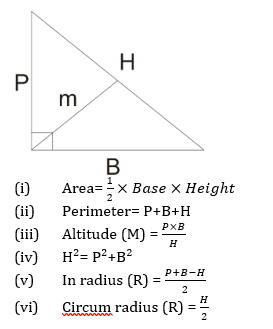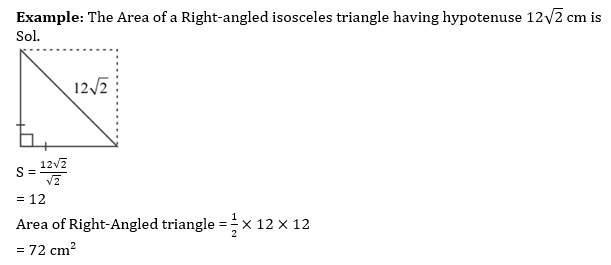• Equilateral triangle: It is a triangle whose all sides and angle are equal.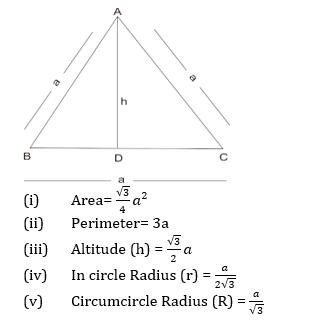• ∠A=∠B=∠C= 60°
• If P₁, P₂, and P₃ are perpendicular lengths from any interior point (O) of an equilateral ∆ ABC to all its three sides respectively, then: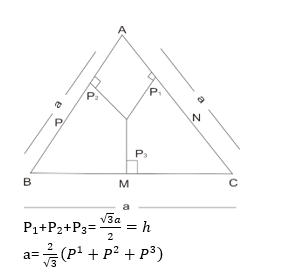Example: Each side of an equilateral triangle is 16 cm. the area of triangle is: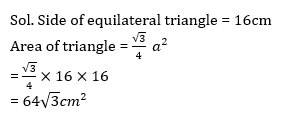Download Mensuration 2D Study Notes PDF in English

Download Mensuration 2D Study Notes PDF in Hindi

Thank You, Your details have been submitted we will get back to you.
•विशेषण- परिभाष�...
•Phases of Teaching - Stages of Teaching ...
•Operant and Classical Conditioning - Dif...
•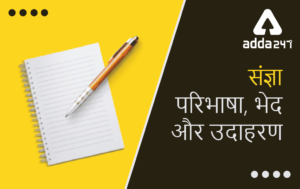संज्ञा: परिभाष�...
•अलंकार - परिभाष�...
•Stages of Development- Child Growth and ...